+关注

2019-08(106)

2019-09(105)

2019-10(7)

2019-11(8)

2019-12(13)

2020-01(23)

2020-02(22)

## Python学习日记(八) 函数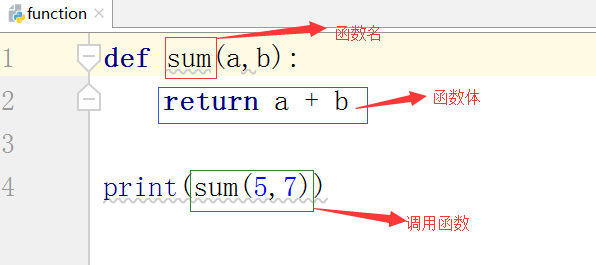1.当函数执行时运到return关键字将不再往下执行

```def func():
print("hello world!")
print("hello world!")
return
print("hello world!")
func()  #hello world!
#hello world!```

2.当函数不写return或者return后面不写值时它的返回值为None

```def func():
print("hello world!")
print(func())   # hello world!
# None                        ```

3.当函数return后面写了一个值就返回该值

4.当函数后面写了多个结果则返回一个元祖,可以通过直接使用元祖来获得多个变量

```def func():
return 1,5,6,7
values = func()
print(values)   #(1, 5, 6, 7)```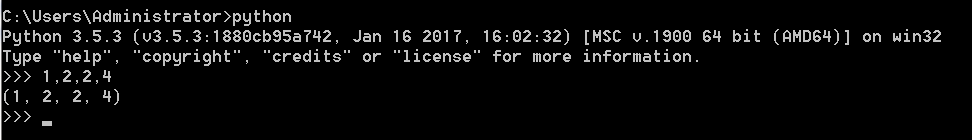python自动将逗号分隔的元素组合成一个元祖，元祖、列表、字典可以进行解包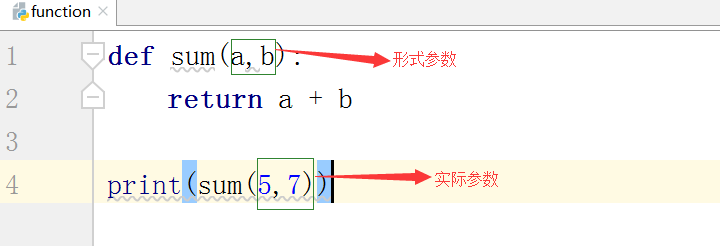<1>形式参数:在函数体使用的参数变量

a.位置参数:按照位置从左到右一一对应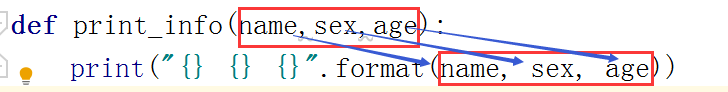b.默认值参数:给函数参数设定一个默认值,例如当我们调用open函数时我们默认的模式就是mode = 'r'，我们就可以省去不写

```def print_info(name,age,sex = 'male'):
print("{} {} {}".format(name, age,sex))
print_info('Jane',age = 15)  #Jane male 15```

```def print_info(name,age,sex = 'male'):
print("{} {} {}".format(name, age,sex))
print_info('Jane',sex = 'female',age = 15)  #Jane female 15```

陷阱:如果默认参数的值是一个可变数据类型,那么每一次调用函数的时候,如果不传值那么就公用这个数据类型的资源

```def func(li = []):
li.append(2)
print(li)
func()      #
func()      #[2, 2]
func([])    #
func()      #[2, 2, 2]```

```def func(dic = {}):
dic['a'] = 'v'
print(dic)

func()      #{'a': 'v'}     key是唯一所以每一次都是覆盖
func()      #{'a': 'v'}
func({})    #{'a': 'v'}
func()      #{'a': 'v'}```
```def func(k,dic = {}):
dic[k] = 'v'
print(dic)
func('a')   #{'a': 'v'}
func('b')   #{'a': 'v', 'b': 'v'
func('c')   #{'a': 'v', 'b': 'v', 'c': 'v'}```

c.动态参数:

①动态接收位置参数:*args 接收所有的位置参数，再以元祖的形式赋予args

```def sum_data(*args):
res = 0
for i in args:
res += i
return res
print(sum_data(1,2,3,4,7,8,-10))    #15```

②动态接收关键字参数:**kwargs 接收所有的关键字参数,再以字典的形式赋予kwargs

```def print_data(**kwargs):
print(kwargs)
print_data(name='Jane',sex='female',age=22)   #{'name': 'Jane', 'sex': 'female', 'age': 22}```

<2>实际参数:在调用函数时使用的参数变量

a.位置参数:从左到右和形参的位置一一对应

```def print_info(name,sex,age):
print("{} {} {}".format(name,sex,age))
print_info('Jane','female',15)  #Jane female 15```

b.关键字参数:当用于繁杂的数据时,不需要记住参数的位置,只需要记住参数的名字即可

```def print_info(name,sex,age):
print("{} {} {}".format(name, sex, age))
print_info(name='Jane',sex='female',age = 15)  #Jane female 15```

c.混合参数:就是位置参数和关键字参数可以混合着使用

原则：当使用这种写法就一定要注意位置参数必须要在关键字参数之前

```def print_info(name,sex,age):
print("{} {} {}".format(name, sex, age))
print_info('Jane','female',age = 15)  #Jane female 15```

*的用法:

1.函数中分为打散和聚合

a.聚合:

当我们定义一个形参为args时,那么这个形参只能接受一个实参,这个实参也就是位置参数

```def func(args):
print(args)

当我们在args前加上一个星号,它可以接收多个参数,并返回一个元祖,**kwargs也有着相同的道理,结果返回一个字典,因此*在函数中有着聚合的作用

b.打散:

将具有可迭代性质的实际参数打散，相当于将这些实际参数给拆解成一个一个的组成元素当成位置参数然后传给args，**起到的是打散的作用

```s = 'hello world!'
li = [1,2,'a']
tu = (10,'p')
def func(*args):
print(args)
func(*s,*li,*tu)    #('h', 'e', 'l', 'l', 'o', ' ', 'w', 'o', 'r', 'l', 'd', '!', 1, 2, 'a', 10, 'p')```

2.函数外可以处理剩下的元素

```# 之前的赋值
a,b = (1,2)
print(a, b)  #1 2
# 用*来处理剩下的元素
c,*d = (1, 2, 3, 4,)
print(c, d)  #1 [2, 3, 4]
*rest, e, f = range(5)
print(rest, e, f)     #[0, 1, 2] 3 4
print([1, 2, *[3, 4, 5]]) # [1, 2, 3, 4, 5]```

1.内置命名空间

a.Python解释器已启动就可以使用的名字，例如print、input、list等存储于内置命名空间中

b.内置的名字在启动解释器时就会被加载进内存里

2.全局命名空间(我们写的代码,但不是函数)

即在程序由上至下执行的过程中依次加载进内存,放置了我们设置的所有变量名和函数名

3.局部命名空间(函数)

a.函数内部定义的名字

b.当调用函数的时候,才会产生这个名称空间,随着函数执行的结束,该命名空间就会被回收了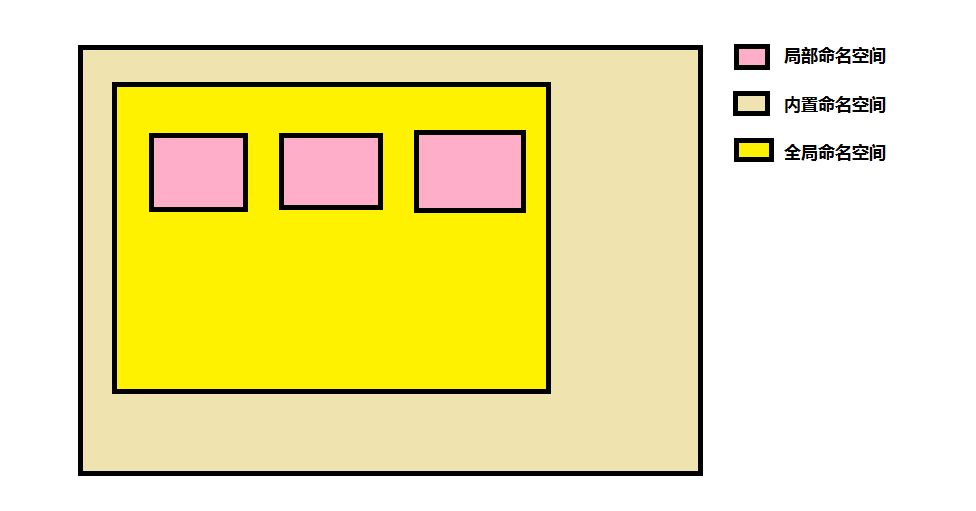```len = 233
def func():
print(len)
return
func()              #233
print(len('aaa'))   #当我们再以内置命名空间中函数的方法去调用就会报错
# TypeError: 'int' object is not callable```

1.全局作用域

作用于全局和内置命名空间,全局和内置命名空间的名称都属于它

2.局部作用域

作用于局部命名空间,局部命名空间(函数)的名称都属于它

global():

```a = 1
def func():
print(a)
func()      #1```

```a = 1
def func():
global a
a += 1
return a
print(func())      #2```

nonlocal()：

```a = 0
def fun1():
a = 1
def fun2():
a = 2
def fun3():
nonlocal a
a += 1
fun3()
print('this a is ',a)
fun2()
print('This a is ',a)

fun1()
print('全局: a =',a)```

a,.只能用于局部变量找上一层离当前函数最近一层的局部变量,声明了nonlocal()的内部函数的变量修改会影响到离当前函数最近一层的局部变量

b.Python 3.x新增功能

c.对全局无效

d.对局部也只是对最近一层有影响

a.globals():以字典的形式返回全局作用域所有的变量对应关系

```a = 1
b = 2
c = 'ass'
print(globals())    #{'__name__': '__main__', '__doc__': None, '__package__': None,
#'__spec__': None, '__annotations__': {}, '__builtins__': <module 'builtins' (built-in)>,
# '__cached__': None, 'a': 1, 'b': 2, 'c': 'ass'}
print(locals())     #打印内容一样```

b.locals():以字典的形式返回当前作用域下所有的变量对应关系

```a = 1
b = 2
def func():
c = 3
d = 'css'
print(globals())    #{'__name__': '__main__', '__doc__': None, '__package__': None,
# '__spec__': None, '__annotations__': {}, '__builtins__': <module 'builtins' (built-in)>,
# '__cached__': None, 'a': 1, 'b': 2, 'func': <function func at 0x00000000028D9730>}
print(locals())     #{'c': 3, 'd': 'css'}
func()```

```def func1(a,b):
return a if a > b else b
def func2(a,b,c):
d = func1(a,b)
return func1(d,c)
print(func2(5,4,3))         #5```

```def print_numbers():
print(456)
def func(f):
f()
return f　　　　　　　　　　　　#函数名作为返回值

func2 = func(print_numbers)     #456　　　　函数名作为参数
func2()                         #456    ```

```def print_int():
print(123456)
print_numbers = print_int   #进行函数赋值
print_numbers()```

```def func1():
print(123)
def func2():
print('abc')
li = [func1,func2]  #将两个函数封装进一个列表
for i in li:
i()```

```def func():
a = 1
def get_int():
print(a)
print(get_int.__closure__)  #(<cell at 0x000000000272C978: int object at 0x000007FEE2ADB350>,)
func()```

```def func():
a = 1
def get1():
name = 'abc'
def get2():
print(a,name)
return get2
return get1
recp = func()
get_recp = recp()
get_recp()```

```def func():
print('hello world!')
print(func)     #<function func at 0x0000000002669730>该函数的内存地址
func()          #函数的调用```

26 0

#### 优质资源排行榜

python经典电子书大合集下载 下载次数 8139

零基础大数据全套视频400G 下载次数 7006

零基础软件测试全套系统教程 下载次数 6506

全套人工智能视频+pdf 下载次数 6443

IOS全套视频教程 基础班+就业班 下载次数 4680

编程小白的第一本python入门书（高清版）PDF下载 下载次数 3654

11  Python深度学习 pdf下载 下载次数 3156

12  笨办法学python pdf下载 下载次数 3088

14  树莓派Python编程指南 pdf下载 下载次数 3011

16  python项目开发视频 下载次数 3002

18  黑马2017年java就业班全套视频教程 下载次数 2992

19  Python基础教程 pdf下载 下载次数 2988

21  利用python实现程序内存监控脚本 下载次数 2987

22  老男孩python自动化视频 下载次数 2983

23  老王python基础+进阶+项目视频教程 下载次数 2974

24  尚硅谷Go学科全套视频 下载次数 2972

25  某硅谷Python项目+AI课程+核心基础视频教程 下载次数 2968

26  Web前端实战精品课程 下载次数 2967

27  Python算法教程_中文版 pdf下载 下载次数 2967

28  tron python小游戏 下载次数 2963

29  [小甲鱼]零基础入门学习Python 下载次数 2962

30  老男孩python全栈开发15期 下载次数 2958

31  2017最新web前端开发完整视频教程附源码 下载次数 2948

32  最新全套完整JAVAWEB2018开发视频 下载次数 2926

33  流畅的Python PDF下载高清完整扫描原版 下载次数 2922

34  Spring boot实战视频6套下载 下载次数 2910

35  Python高性能编程 pdf下载 下载次数 2908

36  python全套视频十五期（116G） 下载次数 2908

37  Python项目实战 下载次数 2887

38  利用Python进行数据分析 pdf下载 下载次数 2885

39  30个小时搞定Python网络爬虫 含源码 下载次数 2884

41  python全自动抢火车票教程-python视频教程下载 下载次数 2883

43  Python A~B~C~ python视频教程下载 下载次数 2866

44  数据结构与算法视频（小甲鱼讲解-全） 下载次数 2864

45  web小程序表白天数倒计时源码下载 下载次数 2863

46  python基础视频教程 下载次数 2862

47  go语言全套视频 下载次数 2855

48  清华学霸尹成Python爬虫视频-ok 下载次数 2846

49  黑马前端36期最全视频和代码 下载次数 2843

50  2018最新全套web前端视频教程+源码下载 下载次数 2842